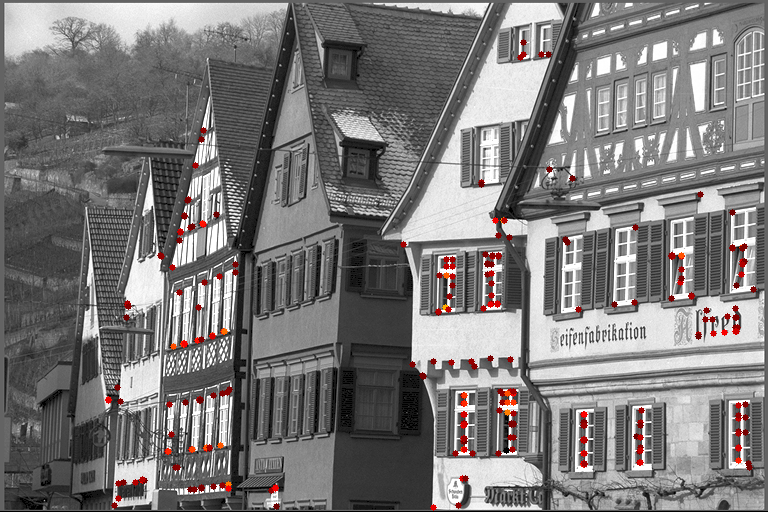## VPI - Vision Programming Interface

#### 2.3 Release

Harris Corner Detector

# Overview

This algorithm implements the Harris keypoint detection operator that is commonly used to detect keypoints and infer features of an image.

The standard Harris detector algorithm as described in  is applied first. After that, a non-max suppression pruning process is applied to the result to remove multiple or spurious keypoints.

Input Parameters Output keypoints\begin{align*} \mathit{gradientSize} &= 5 \\ \mathit{blockSize} &= 5 \\ \mathit{strengthThresh} &= 20 \\ \mathit{sensitivity} &= 0.01 \\ \mathit{minNMSDistance} &= 8 \end{align*}# Implementation

1. Compute the spatial gradient of the input using one of the following filters, depending on the value of VPIHarrisCornerDetectorParams::gradientSize :

\begin{align*} \mathit{sobel}_x &= \frac{1}{4} \cdot \begin{bmatrix} 1 \\ 2 \\ 1 \end{bmatrix} \cdot \begin{bmatrix} -1 & 0 & 1 \end{bmatrix} \\ \mathit{sobel}_y &= (\mathit{sobel}_x)^\intercal \end{align*}

\begin{align*} \mathit{sobel}_x &= \frac{1}{16} \cdot \begin{bmatrix} 1 \\ 4 \\ 6 \\ 4 \\ 1 \end{bmatrix} \cdot \begin{bmatrix} -1 & -2 & 0 & 2 & 1 \end{bmatrix} \\ \mathit{sobel}_y &= (\mathit{sobel}_x)^\intercal \end{align*}

\begin{align*} \mathit{sobel}_x &= \frac{1}{64} \cdot \begin{bmatrix} 1 \\ 6 \\ 15 \\ 20 \\ 15 \\ 6 \\ 1 \end{bmatrix} \cdot \begin{bmatrix} -1 & -4 & -5 & 0 & 5 & 4 & 1 \end{bmatrix} \\ \mathit{sobel}_y &= (\mathit{sobel}_x)^\intercal \end{align*}

2. Compute a gradient covariance matrix (structure tensor) for each pixel within a block window, as described by:

$M = \sum_{p \in B}\begin{bmatrix}I_x^2(p) & I_x(p) I_y(p) \\ I_x(p) I_y(p) & I_y^2(p) \end{bmatrix}$

where:

• p is a pixel coordinate within B, a block window of size 3x3, 5x5 or 7x7.
• $$I(p)$$ is the input image
• $$I_x(p) = I(p) * \mathit{sobel}_x$$
• $$I_y(p) = I(p) * \mathit{sobel}_y$$
3. Compute a Harris response score using a sensitivity factor

$R = \mathit{det}(M) - k \cdot \mathit{trace}^2(M )$

where k is the sensitivity factor

4. Applies a threshold-strength criterion, pruning keypoints whose response <= VPIHarrisCornerDetectorParams::strengthThresh.
5. Applies a non-max suppression pruning process.

This process splits the input image into a 2D cell grid. It selects a single corner with the highest response score inside the cell. If several corners within the cell have the same response score, it selects the bottom-right corner.

# C API functions

For list of limitations, constraints and backends that implements the algorithm, consult reference documentation of the following functions:

Function Description
vpiInitHarrisCornerDetectorParams Initializes VPIHarrisCornerDetectorParams with default values.
vpiCreateHarrisCornerDetector Creates a Harris Corner Detector payload.
vpiSubmitHarrisCornerDetector Submits a Harris Corner Detector operation to the stream.

# Usage

Language:
1. Import VPI module
import vpi
2. Execute the algorithm on the input image using the CUDA backend. Two VPI arrays are returned, one with keypoint positions, and another with the scores. The keypoints array has type vpi.Type.KEYPOINT and scores array has type vpi.Type.U32.
with vpi.Backend.CUDA:
keypoints, scores = input.harriscorners(sensitivity=0.01)
1. Initialization phase
1. Include the header that defines the Harris corner detector function.
Declares functions that implement the Harris Corner Detector algorithm.
2. Define the input image object.
VPIImage input = /*...*/;
struct VPIImageImpl * VPIImage
A handle to an image.
Definition: Types.h:256
3. Create the output arrays that will store the keypoints and their scores.
VPIArray keypoints;
VPIArray scores;
vpiArrayCreate(8192, VPI_ARRAY_TYPE_U32, 0, &scores);
VPIStatus vpiArrayCreate(int32_t capacity, VPIArrayType type, uint64_t flags, VPIArray *array)
Create an empty array instance.
struct VPIArrayImpl * VPIArray
A handle to an array.
Definition: Types.h:232
@ VPI_ARRAY_TYPE_U32
Unsigned 32-bit.
Definition: ArrayType.h:76
@ VPI_ARRAY_TYPE_KEYPOINT_F32
VPIKeypointF32 element.
Definition: ArrayType.h:77
4. Since this algorithm needs temporary memory buffers, create the payload for it on the CUDA backend.
int32_t w, h;
vpiImageGetSize(input, &w, &h);
Creates a Harris Corner Detector payload.
VPIStatus vpiImageGetSize(VPIImage img, int32_t *width, int32_t *height)
Get the image dimensions in pixels.
A handle to an algorithm payload.
Definition: Types.h:268
@ VPI_BACKEND_CUDA
CUDA backend.
Definition: Types.h:93
5. Create the stream where the algorithm will be submitted for execution.
VPIStream stream;
vpiStreamCreate(0, &stream);
struct VPIStreamImpl * VPIStream
A handle to a stream.
Definition: Types.h:250
VPIStatus vpiStreamCreate(uint64_t flags, VPIStream *stream)
Create a stream instance.
2. Processing phase
1. Initialize the configuration structure with default parameters and set sensitivity to a new value.
params.sensitivity = 0.01;
float sensitivity
Specifies sensitivity threshold from the Harris-Stephens equation.
Definition: HarrisCorners.h:96
VPIStatus vpiInitHarrisCornerDetectorParams(VPIHarrisCornerDetectorParams *params)
Initializes VPIHarrisCornerDetectorParams with default values.
Structure that defines the parameters for vpiSubmitHarrisCornerDetector.
Definition: HarrisCorners.h:82
2. Submit the algorithm and its parameters to the stream. It'll be executed by the CUDA backend associated with the payload.
vpiSubmitHarrisCornerDetector(stream, 0, harris, input, keypoints, scores, &params);
VPIStatus vpiSubmitHarrisCornerDetector(VPIStream stream, uint64_t backend, VPIPayload payload, VPIImage input, VPIArray outFeatures, VPIArray outScores, const VPIHarrisCornerDetectorParams *params)
Submits a Harris Corner Detector operation to the stream.
3. Optionally, wait until the processing is done.
vpiStreamSync(stream);
VPIStatus vpiStreamSync(VPIStream stream)
Blocks the calling thread until all submitted commands in this stream queue are done (queue is empty)...
3. Cleanup phase
1. Free resources held by the stream, the payload, the input image and the output arrays.
vpiArrayDestroy(keypoints);
vpiArrayDestroy(scores);
void vpiArrayDestroy(VPIArray array)
Destroy an array instance.
void vpiImageDestroy(VPIImage img)
Destroy an image instance.
Deallocates the payload object and all associated resources.
void vpiStreamDestroy(VPIStream stream)
Destroy a stream instance and deallocate all HW resources.

For more information, see Harris Corners in the "C API Reference" section of VPI - Vision Programming Interface.

# Performance

For information on how to use the performance table below, see Algorithm Performance Tables.
Before comparing measurements, consult Comparing Algorithm Elapsed Times.
For further information on how performance was benchmarked, see Performance Benchmark.

-

# References

1. C. Harris, M. Stephens (1988), "A Combined Corner and Edge Detector"
Proceedings of Alvey Vision Conference, pp. 147-151.